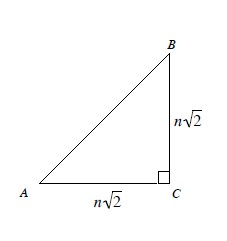### Home > PC > Chapter 3 > Lesson 3.3.1 > Problem3-112

3-112.

Using $\triangle ABC$ below to complete the following problems.1. Find the exact length of $\overline{A B}$ in terms of $n$.

$AB^2 = (n\sqrt{2})^2 + (n\sqrt{2})^2$

$AB^2 = 2n^2+2n^2$

2. In terms of $n$, find $\sin A$.

$\sin(A) = \frac{\text{opposite}}{\text{hypotenuse}}$

3. If $n = 12$, what is the value of $\sin A$?

${\frac{\sqrt{2}}{2}}$

4. What does this tell you about $\sin45^\circ$?

It does not matter what the side length is as long as $A = 45^\circ$.

5. What about $\cos45^\circ$?

$\cos\left(45^{\circ}\right)=\sin\left(45^{\circ}\right)$